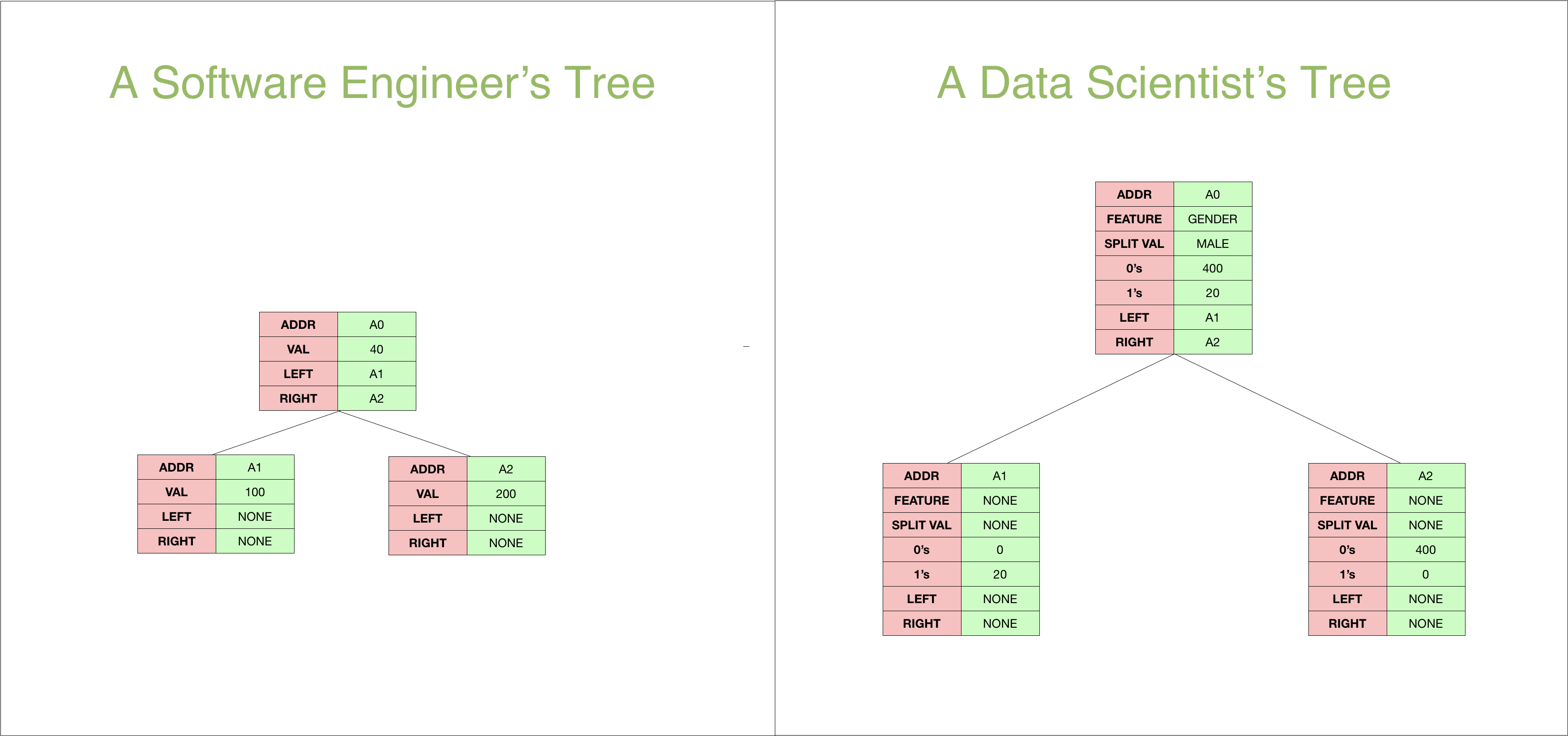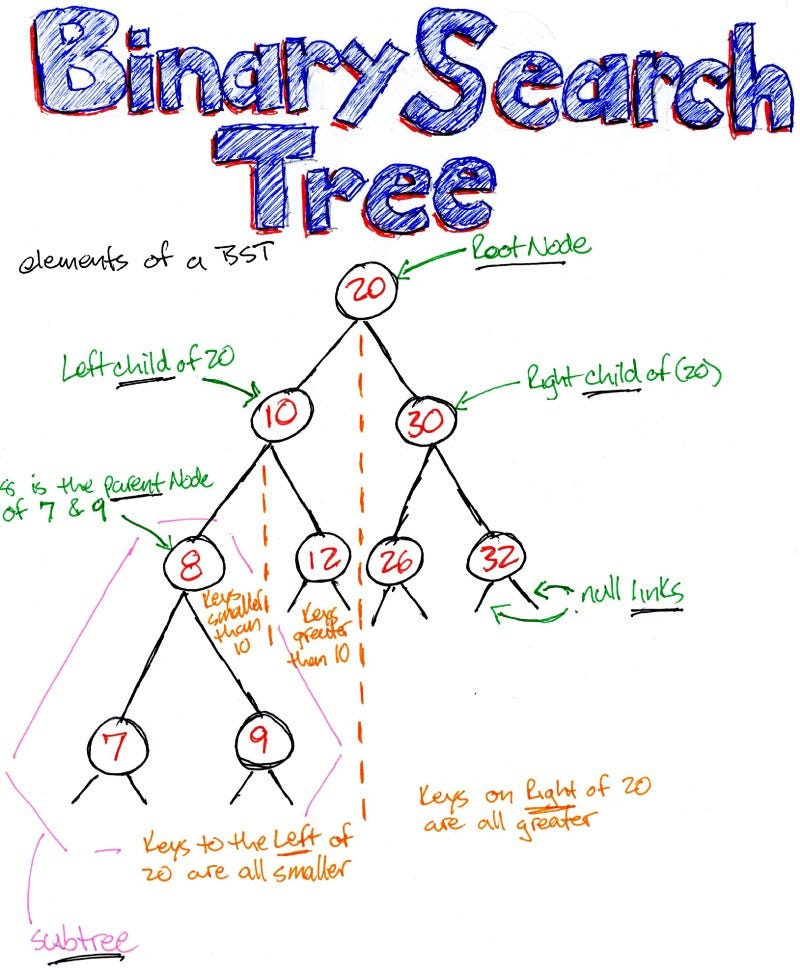Topics: AI | Data Science | Data Visualization | Deep Learning | Machine Learning | NLP | Python | R | Statistics

KDnuggets Home » News » 2020 » Jan » Tutorials, Overviews » Handling Trees in Data Science Algorithmic Interview ( 20:n03 )

# Handling Trees in Data Science Algorithmic Interview

This post is about fast-tracking the study and explanation of tree concepts for the data scientists so that you breeze through the next time you get asked these in an interview.

Algorithms and data structures are an integral part of data science. While most of us data scientists don't take a proper algorithms course while studying, they are crucial all the same.

Many companies ask data structures and algorithms as part of their interview process for hiring data scientists.

Now the question that many people ask here is what is the use of asking a data scientist such questions. The way I like to describe it is that a data structure question may be thought of as a coding aptitude test.

We all have given aptitude tests at various stages of our life, and while they are not a perfect proxy to judge someone, almost nothing ever really is. So, why not a standard algorithm test to judge people's coding ability.

But let's not kid ourselves, they will require the same zeal to crack as your Data Science interviews, and thus, you might want to give some time for the study of algorithms and Data structure questions.

This post is about fast-tracking this study and explaining tree concepts for the data scientists so that you breeze through the next time you get asked these in an interview.

### But First, Why are Trees important for Data Science?

To data scientists, Trees mean a different thing than they mean for a Software Engineer.

For a software engineer, a tree is just a simple Data Structure they can use to manage hierarchical relationships while for a Data Scientists trees form the basis of some of the most useful classification and regression algorithms.

So where do these two meet?

They are necessarily the same thing. Don't be surprised. Below is how data scientists and software engineer's look at trees.They are essentially the same

The only difference is that Data science tree nodes keep much more information that helps us in identifying how to traverse the tree. For example, in the case of Data science tree for prediction, we will look at the feature in the node and determine which way we want to move based on the split value.

If you want to write your decision tree from scratch, you might need to understand how trees work from a software engineering perspective too.

### Types of Trees:

In this post, I will only be talking about two kinds of trees that get asked a lot in Data Science interview questions. Binary Trees(BT) and an extension of Binary Trees called Binary Search Trees(BST).

### 1. Binary Trees:

A binary tree is simply a tree in which each node has up to two children. A decision tree is an example we see in our day to day lives.Binary Tree: Each Node has up to 2 children

### 2. Binary Search Tree(BST):

A binary search tree is a binary tree in which:

• All left descendants of a node are less than or equal to the node, and
• All right descendants of the node are greater than the node.

There are variations to this definition when it comes to equalities. Sometimes the equalities are on the right-hand side or either side. Sometimes only distinct values are allowed in the tree.8 is greater than all the elements in the left subtree and smaller than all elements in the right subtree. The same could be said for any node in the tree.

### Creating a Simple Tree:

So How do we construct a simple tree?

By definition, a tree is made up of nodes. So we start by defining the `node` class which we will use to create nodes. Our node class is pretty simple as it holds value for a node, the location of the left child and the location of the right child.

```class node:
def __init__(self,val):
self.val = val
self.left = None
self.right = None```

We can create a simple tree now as:

```root = node(1)
root.left = node(2)
root.right = node(3)```

Now I have noticed that we cannot really get the hang of Tree-based questions without doing some coding ourselves.

So let us get a little deeper into the coding part with some problems I found most interesting when it comes to trees.

### Inorder Tree Traversal:

There are a variety of ways to traverse a tree, but I find the inorder traversal to be most intuitive.

When we do an inorder traversal on the root node on a Binary Search tree, it visits/prints the node in ascending order.

```def inorder(node):
if node:
inorder(node.left)
print(node.val)
inorder(node.right)```

This above method is pretty important as it allows us to visit all the nodes.

So if we want to search for a node in any binary tree, we might try to use inorder tree traversal.

### Creating a Binary Search Tree from a Sorted array

What kind of coders will we be if we need to create a tree piece by piece manually as we did above?

So can we create a BST from a sorted array of unique elements?

```def create_bst(array,min_index,max_index):
if max_index<min_index:
return None
mid = int((min_index+max_index)/2)
root = node(array[mid])
leftbst = create_bst(array,min_index,mid-1)
rightbst = create_bst(array,mid+1,max_index)
root.left = leftbst
root.right = rightbst
return root
a = [2,4,5,6,7]
root = create_bst(a,0,len(a)-1)```

Trees are inherently recursive, and so we use recursion here. We take the mid element of the array and assign it as the node. We then apply the `create_bst` function to the left part of the array and assign it to `node.left` and do the same with the right part of the array.

And we get our BST.

Have we done it right? We can check it by creating the BST and then doing an inorder traversal.

```inorder(root)
------------------------------------------------------------
2
4
5
6
7```

Seems Right!

### Let's check if our tree is a Valid BSTThink Recursion!!!

But again what sort of coders are we if we need to print all the elements and check manually for the BST property being satisfied?

Here is a simple code to check if our BST is valid or not. We assume strict inequality in our Binary Search Tree.

```def isValidBST(node, minval, maxval):
if node:
# Base case
if node.val<=minval or node.val>=maxval:
return False
# Check the subtrees changing the min and max values
return isValidBST(node.left,minval,node.val) &   isValidBST(node.right,node.val,maxval)
return True
isValidBST(root,-float('inf'),float('inf'))
--------------------------------------------------------------
True```

We check the subtrees recursively if they satisfy the Binary Search tree property or not. At each recursive call, we change the `minval` or `maxval` for the call to provide the function with the range of allowed values for the subtree.

### Conclusion

In this post, I talked about Trees from a software engineering perspective. If you want to see trees from a data science perspective, you might take a look at this post.

The Simple Math behind 3 Decision Tree Splitting criterions
Understanding Splitting Criterions

Trees form the basis of some of the most asked questions in Data Science algorithmic interviews. I used to despair such tree-based questions in the past, but now I have grown to like the mental exercise involved in them. And I love the recursive structure involved in such problems.

And while you can go a fair bit in data science without learning them, you can learn them just for a little bit of fun and maybe to improve your programming skills.

Here is a small notebook for you where I have put all these small concepts for you to try and run.
Take a look at my other posts in the Algorithmic Interviews Series, if you want to learn about RecursionDynamic Programming or Linked Lists.

### Continue Learning

If you want to read up more on Algorithms and Data structures, here is an Algorithm Specialization on Coursera by UCSanDiegowhich I highly recommend.

Thanks for the read. I am going to be writing more beginner-friendly posts in the future too. Follow me up at Medium or Subscribe to my blog to be informed about them. As always, I welcome feedback and constructive criticism and can be reached on Twitter @mlwhiz.

Also, a small disclaimer: There might be some affiliate links in this post to relevant resources, as sharing knowledge is never a bad idea.

Bio: Rahul Agarwal is Senior Statistical Analyst at WalmartLabs. Follow him on Twitter @mlwhiz.

Original. Reposted with permission.

Related:

Top Stories Past 30 Days
Most Popular
Most SharedGet KDnuggets, a leading newsletter on AI, Data Science, and Machine Learning